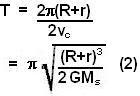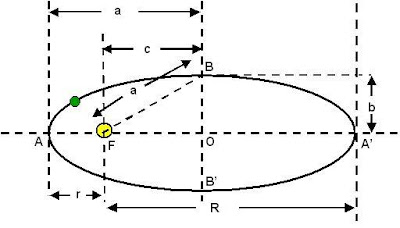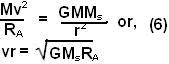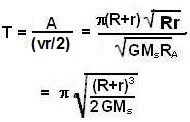## Tuesday, August 26, 2008

### Irodov Problem 1.202

The problem can be solved using both third or the second law. I shall solve the problem using both methods.

Solution using Kepler's third law:
"The squares of the orbital periods of planets are directly proportional to the cubes of the semi-major axis of the orbits."

As the planet travels along the elliptic path its tangential velocity changes from point to point and this makes it a bit hard to compute the exact time period mathematically. From the third law, however, it is clear that the period only depends on the length of the semi-major axis of the path. In other words all elliptical paths with the same semi-major axis will have the same period, the simplest being the circle. So it is sufficient to find the period of the planet in a circular orbit of radius (semi-major axis of the circle) R+r/2. What makes this easier than the elliptic orbit is the fact that in a circular orbit the tangential velocity of the planet will be constant unlike in case of the ellipse.

Let us now consider a planet moving in a circular path with radius (R+r)/2 and find its period.
Since at each point the gravitational radial force accelerates the planet this must provide for the centripetal acceleration of the planet. So if the tangential velocity of planet along this circular path is vc, and the mass of the sun is Ms then we have,The path traveled by the planet is the circumference of the circle (2*pi*(r+R)/2) and the orbital time can be determined by dividing it by the tangential velocity of the planet along the circular path. Hence we have,Solution using Kepler's second law:
"A line joining a planet and the sun sweeps out equal areas during equal intervals of time."In other words the rate at which the planet sweeps the area of the ellipse is always the same no matter at what point of the orbit the planet lies. So if we divide the total area of the ellipse by this rate of area sweep we can find the time taken for the planet to orbit!!

The planet moves along the ellipse shown in the figure.
The sun lies at the focus F. The closest and farthest approaches of the planet are at the ends of major axis A and A'. As in the problem AF=r, A'F=R. The center of the ellipse is at O. It is elementary to see that BF = a = (R+r)/2, OF = c = (R-r)/2. We can now derive OB = b as,Some properties of the ellipse are conveniently provided at this site and will be useful in solving this problem. The area of the ellipse is then given by,Now let us compute the rate at which the planet sweeps the area. We shall compute the instantaneous rate of area swept by the planet at point A, since this is one of the easiest points to do so. Suppose that the planet move an infinitesimally small distance AD of length dy along the tangent to the ellipse at point A. The infinitesimally small area of the ellipse swept the by the planet is area of the triangle ADF given by rdy/2 (half of the area ADD'F). If this is done in an infinitesimally small time dt, then the rate of the sweep of the area is given by, where v is instantaneous tangential velocity of the planet at point A.Now let us compute the instantaneous velocity of the planet at point A. This can be done from the fact that the radial gravitational force at each point at the ellipse provide for the centripetal acceleration of the planet. The instantaneous centripetal acceleration of the planet at point A is given by v*v/RA , where RA is the radius of curvature of the elliptic path at point A (see the site) given by,Hence, we have,Now this is also the rate at which area is being swept. So we have the time period as,Isn't it cool that we arrived at the same result using either of the laws!!Rupinder Singh said...

sir, I have a doubt in prob.1.202. I could not understand the concept of radius of curvature.
I would be greatly obliged if you help clearing my doubt please.

Krishna Kant Chintalapudi said...

When a particle moves along any other curve other than a circle, where does one say the center is? Basically you have to find a circle to which the instantenous direction of motion is a tangent. The center of this circle must lie on the perpendicular to the tangent. Then we need to find the center on this perpendicular line v = wr. r is then the radius of curvature.

Dantephysics said...

Sir,
Up till now this was the coolest solution I have ever seen on your site(the second part).
Thank you Sir!